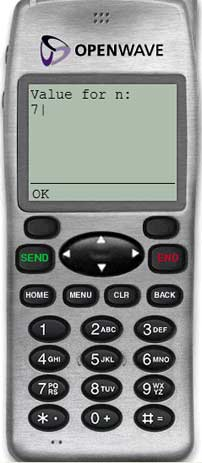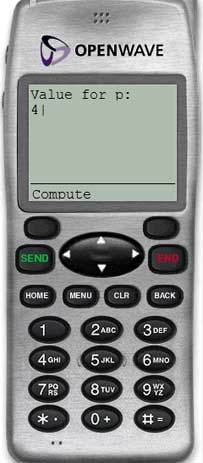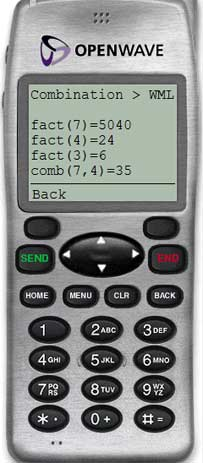Home > Phone > Samples > Combination > WML and WMLScriptCombination in WML/WMLScript

####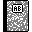Purpose

This is the WAP version of our combination application. It uses WML and WMLScript.

Your phone speaks WAP? Great! Point it at mobile.eric-poncet.com/phone/samples/combination.wml.

####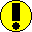Requirements

You need a phone emulator that is WMLScript-compliant, such as OpenWave (see tools section).

####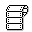Source code

Below is the .wml file, which is the GUI of our application.

```<?xml version="1.0"?>
<!DOCTYPE wml PUBLIC "-//WAPFORUM//DTD WML 1.1//EN" "http://www.wapforum.org/DTD/wml_1.1.xml">
<!--
Combination.wml
Cell phone
WML/WMLScript
-->

<wml>

<card id="input" >
<onevent type="onenterforward">
<refresh>
<setvar name="n" value=""/>
<setvar name="p" value=""/>
<setvar name="n_p" value=""/>
<setvar name="factorialN" value=""/>
<setvar name="factorialP" value=""/>
<setvar name="factorialN_P" value=""/>
<setvar name="combinationNP" value=""/>
</refresh>
</onevent>

<p>
<do type="accept" label="Compute">
<go href="combination.wmls#go()"/>
</do>

Value for n:
<input type="text" name="n" format="*N"/>
Value for p:
<input type="text" name="p" format="*N"/>
</p>
</card>

<card id="result">
<p>
<do type="accept" label="Back">
<go href="#input">
<setvar name="n" value=""/>
<setvar name="p" value=""/>
</go>
</do>

Combination &gt; WML<br/>
<br/>
fact(\$n)=\$factorialN
fact(\$p)=\$factorialP
fact(\$n_p)=\$factorialN_P
comb(\$n,\$p)=\$combinationNP
</p>
</card>
</wml>
```

And here's the .wmls file, which contains the logic of our app.

```//Combination.wmls
//Cell phone
//WML/WMLScript

extern function go()
{
var strN = WMLBrowser.getVar("n"),
strP = WMLBrowser.getVar("p");
var n = Lang.parseInt(strN),
p = Lang.parseInt(strP),
result;

if (n > 0)
if (p > 0)
if (n >= p)
{
WMLBrowser.setVar("n_p", n-p);
WMLBrowser.setVar("factorialN", factorial(n));
WMLBrowser.setVar("factorialP", factorial(p));
WMLBrowser.setVar("factorialN_P", factorial(n-p));
WMLBrowser.setVar("combinationNP", combination(n, p));
WMLBrowser.go("combination.wml#result");
}
else error("'p' > 'n'");
else error("Invalid value for 'p'");
else error("Invalid value for 'n'");
}

function factorial(n)
{
return (n <= 1) ? 1 : n*factorial(n-1); // he-he... let's mix a ternary operator (?) and recursion...
}

function combination(n, p)
{
return factorial(n) div (factorial(n - p) * factorial(p));
}

function error(msg)
{
WMLBrowser.setVar("n", "");
WMLBrowser.setVar("p", "");
WMLBrowser.go("#input");
}
```

####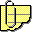Comments

The .wml deck contains two cards:

• input is a form allowing the user to input values for 'n' and 'p' and launch the computation
• result displays the results

The .wmls contains the logic of our applications, that is, the computation of factorial and combination.

Also, we included a consitency check within the logic, by making sure that both n and p are not null or zero, and that n is greater than or equal to p. Here's what the user gets if s/he enters numbers that do not comply with those rules: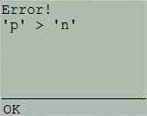The core functions are located in the .wmls file: factorial(), combination() and go(). Only go() can be called from WML, as it's the only extern function.